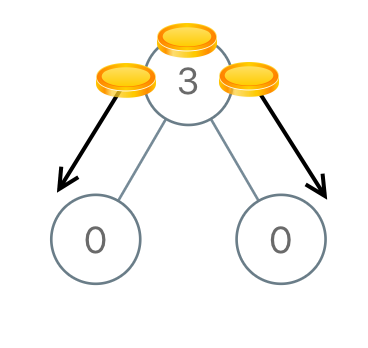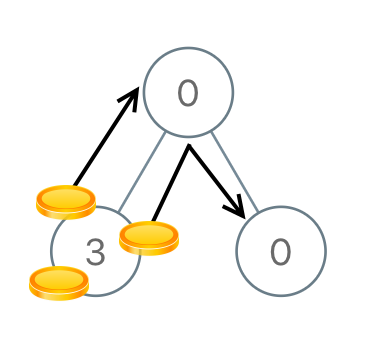979. Distribute Coins in Binary Tree
Medium
4.6K
152

You are given the `root` of a binary tree with `n` nodes where each `node` in the tree has `node.val` coins. There are `n` coins in total throughout the whole tree.

In one move, we may choose two adjacent nodes and move one coin from one node to another. A move may be from parent to child, or from child to parent.

Return the minimum number of moves required to make every node have exactly one coin.

Example 1:```Input: root = [3,0,0]
Output: 2
Explanation: From the root of the tree, we move one coin to its left child, and one coin to its right child.
```

Example 2:```Input: root = [0,3,0]
Output: 3
Explanation: From the left child of the root, we move two coins to the root [taking two moves]. Then, we move one coin from the root of the tree to the right child.
```

Constraints:

• The number of nodes in the tree is `n`.
• `1 <= n <= 100`
• `0 <= Node.val <= n`
• The sum of all `Node.val` is `n`.
Accepted
102.4K
Submissions
141.7K
Acceptance Rate
72.3%

Seen this question in a real interview before?
1/4
Yes
No

Discussion (0)

Related Topics